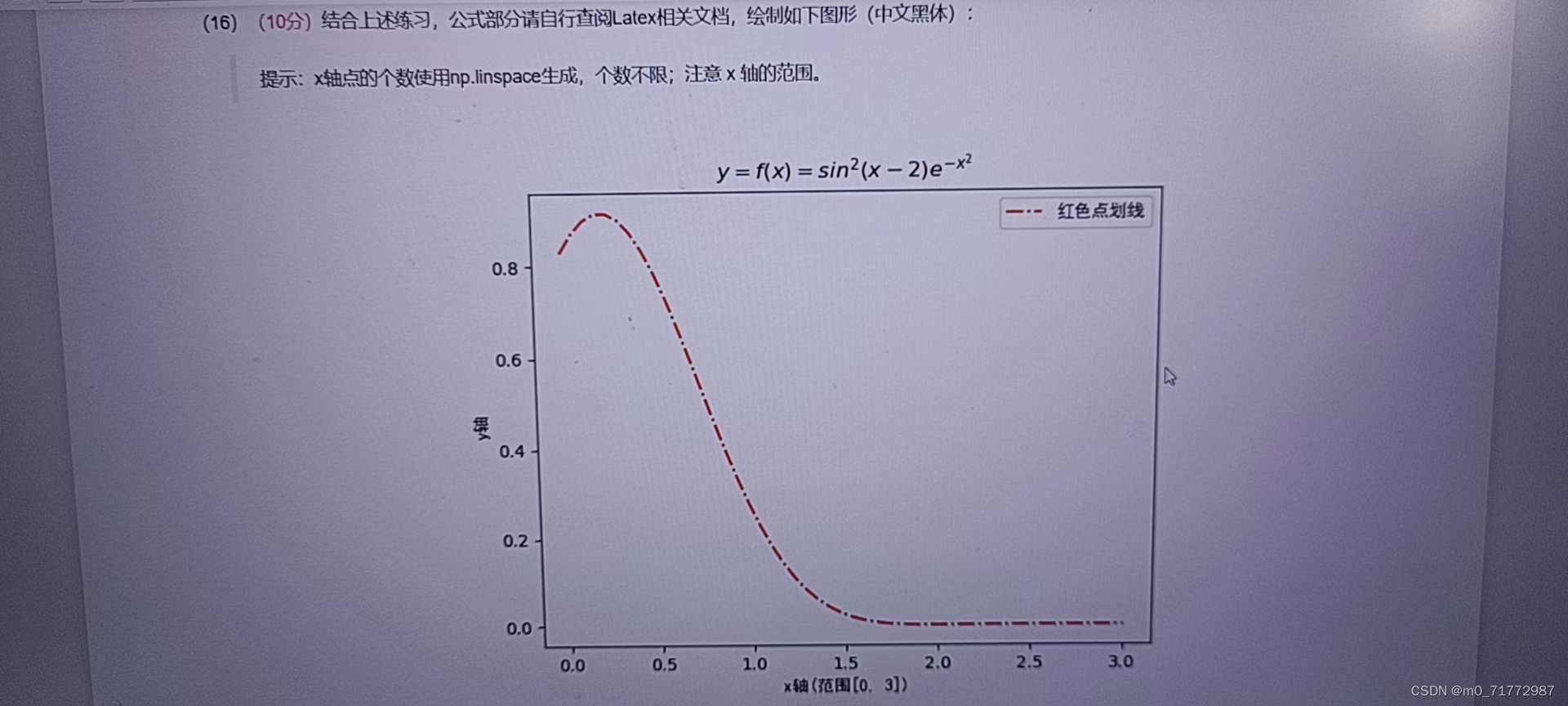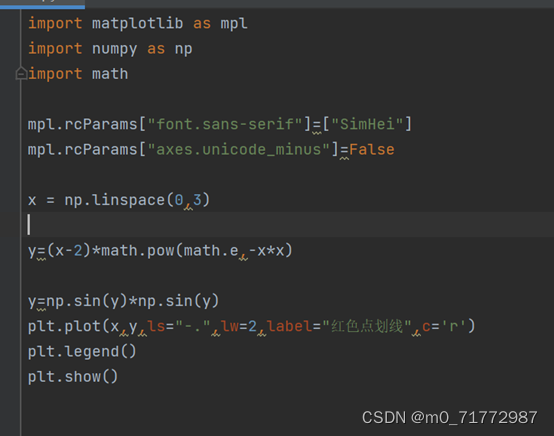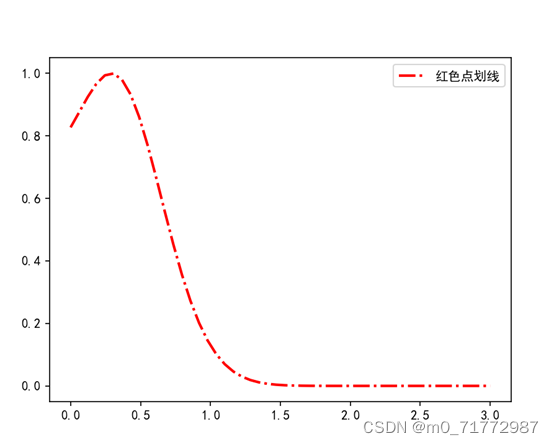# TypeError: only size-1 arrays can be converted to Python scalars 报错如何解决TypeError: only size-1 arrays can be converted to Python scalarsimport matplotlib.pyplot as plt

import matplotlib as mpl
import numpy as np
import math

mpl.rcParams["font.sans-serif"]=["SimHei"]
mpl.rcParams["axes.unicode_minus"]=False

x = np.linspace(0,3)
a=[]
for i in x:
k=(i-2)*math.pow(math.e,-i*i)
a.append(k)
y=np.sin(a)*np.sin(a)
plt.plot(x,y,ls="-.",lw=2,label="红色点划线",c='r')
plt.legend()
plt.show()